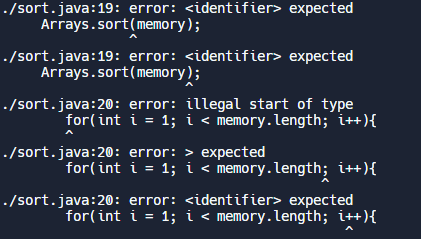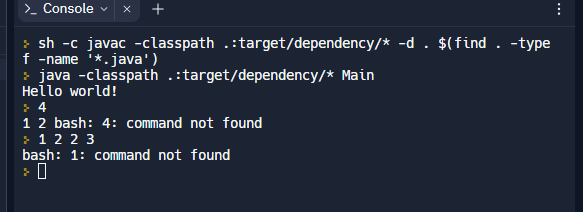# Debugging Help CodeForces Problem

## Checklist

Make sure to read all of How to ask for help on a problem before asking for help.

Cereal Sort

## Question

I’m not sure why I’m getting the error that my statement at line 14 is unreachable.

Also this isn’t as high of a priority, but how would I go about decreasing the Big O value of this?

## What I’ve Tried

Before this, my error was with not finding a variable, so I inserted a print statement at the end of the loop to figure out what was going wrong, and that’s when I got the above error.

## My Work

``````import java.util.Scanner;
import java.util.Arrays;
import java.util.ArrayList;
import java.util.Collections;

public class sort{
public static void Main(String[] args){
ArrayList<Integer> memory = new ArrayList<Integer>();
while(true)
{
}
Collections.sort(memory);
for(int i = 0; i < memory.size() ; i++){
int k = memory.size();
int min = memory.get(0);
ArrayList<Integer> mem2 = new ArrayList<Integer>();
memory.removeAll(Collections.singleton(min));
k = k + memory.size();
System.out.println(k);
}
//System.out.println(k);
}
}

``````

I’m creating an arraylist using the input from the user, sorting it, taking the minimum value and putting it in a new arraylist, finding the size of the resulting array, and then repeating until the first array is empty. Ultimately, I want to output the sum of the sizes of the first array.

I’m still relatively new to this so any advice would be really appreciated!

Hello coder_sp,
I’m pretty sure that the ‘unreachable code’ error is because you wrote an infinite loop on line 10: `while(true) {...}`
The loop will run forever and ever, so any code below it cannot run. To fix this, I would first read and store the value of `n` (the number of red pandas), and then loop `n` times to insert the ids.
You mentioned wanting to decrease the Big O of this, so for starters I would recommend using a plain old regular array for the memory. The number of red pandas in the line is already given, so you know the size of the array beforehand and therefore can use it without any problems. `ArrayList` is slow when adding elements.
Could you explain what you’re doing inside of the loop on line 15? The size of `memory` seems to be changing as you’re iterating through it, which could be problematic.
Also, the value of k is not visible outside of the for loop, since you defined it inside the loop and it has block scope.
Hope this helps!Ok so here’s my revised code with the ArrayList changed to an array, while loop changed and variables declared outside the loops.

On line 15, I was using the loop to add the elements on line 2 to the array so that was my intention… Is there a better way to do that?
Heres my new code:

``````import java.util.Scanner;
import java.util.Arrays;
import java.util.ArrayList;
import java.util.Collections;

public class sort{
public static void main(String[] args){
Scanner r = new Scanner(System.in);
int n = r.nextInt();
int memory[];
memory = new int[n];
for (int i = 0; i < n; i++)

}
int k;
Arrays.sort(memory);
for(int i = 1; i < memory.length; i++){
k = memory.length;
int min = memory;
int mem2[];
mem2 = new int[n];
memory.toString();
removeElement(memory, min);
k = k + memory.length;
System.out.println(k);
}
//System.out.println(k);

@Override
public String toString() {
return "sort []";
}
public static int removeElement(int[] nums, int elem) {
int length = nums.length;
if(length==0) return 0;
int i=0;
for(int j=0; j<length; j++)
{
if(nums[j]!=elem)
{
nums[i]=nums[j];
i++;
}
}
if(i<length) nums[i]='\0';
return i;
}
public static int[] addX(int n, int arr[], int x)
{
int j;

// create a new array of size n+1
int newarr[] = new int[n + 1];

// insert the elements from
// the old array into the new array
// insert all elements till n
// then insert x at n+1
for (j = 0; j < n; j++)
newarr[j] = arr[j];

newarr[n] = x;

return newarr;
}
}

``````

These are the errors I face now:Regarding the errors, check the first `for` loop in the code very carefully.

Updated code. Now it’s not taken in the user input for some reason.

Really sorry about all the questions but I genuinely can’t see what I’m doing wrong.

``````import java.util.Scanner;
import java.util.Arrays;
import java.util.ArrayList;
import java.util.Collections;

public class sort{
public static void main(String[] args){
Scanner sc = new Scanner(System.in);
int x= sc.nextInt();
System.out.println(x);

Scanner r = new Scanner(System.in);
int n = r.nextInt();
int memory[];
memory = new int[n];
for (int i = 0; i < n; i++){
//        System.out.println("PLease enter the array element");
int k;
Arrays.sort(memory);
for(int i = 1; i < memory.length; i++){
k = memory.length;
int min = memory;
int mem2[];
mem2 = new int[n];
memory.toString();
removeElement(memory, min);
k = k + memory.length;
System.out.println(k);
}
//System.out.println(k);
r.close();
}

static int removeElement(int[] nums, int elem) {
int length = nums.length;
if(length==0) return 0;
int i=0;
for(int j=0; j<length; j++)
{
if(nums[j]!=elem)
{
nums[i]=nums[j];
i++;
}
}
if(i<length) nums[i]='\0';
return i;
}

public static int[] addX(int n, int arr[], int x)
{
int j;

// create a new array of size n+1
int newarr[] = new int[n + 1];

// insert the elements from
// the old array into the new array
// insert all elements till n
// then insert x at n+1
for (j = 0; j < n; j++)
newarr[j] = arr[j];

newarr[n] = x;

return newarr;*/
}
}
``````

The questions are fine - I’m a beginner too so I feel you ._.
Looks like you made three `Scanner` objects - only one is needed for I/O stuff.
Are you testing your code on Replit? Because then the console will wait for you, the user, to input the information. So to make it work you could just enter in the lines manually by yourself, and it should work.

Ok, I understand the second for loop now, and I can suggest ways to optimize/debug it. First, make sure you initialize `k`, `mem2,` and `min` outside of the loop so their values don’t get reset after every iteration. `i` should also start from 0, not 1. It looks like you’re assigning mem2 to an array of size n+1, though mem2 is of size n and that could cause an error. Instead of iterating through `memory`, though, have you thought about just keeping track of how many id’s there are of each? For example, if the id’s are stored like this: `{1, 3, 3, 1, 2, 1}`, then the sorting algorithm would work like this:

1. Scan through all 6 elements
2. 3 of them are ones, so the sorter will remove 3 pandas from the first line, leaving the other 3 in the line.
3. Scan through all 3 elements
4. 1 of them is a two, so the sorter will remove 1 panda from the first line, leaving 2 in the line.
5. Scan through all 2 elements
6. 2 of them are threes, so the sorter will remove 2 p<andas and leave 0 in the line
Notice that it doesn’t matter if you simulate the the process or not - the actual thing that matters here is keeping track of how many red pandas have each id.

Ok so I’ve deleted the Scanners, and yes I am using replit (which is definitely an improvement from the USACO guide ide and eclipse which I’d previously been using!).

I’ve initialized the variables outside the for loop, so hoping that fixes things.

No I haven’t but that sounds like a much easier approach. I haven’t used anything like this before, but here’s the updated code I came up with.
Basically, I made a new method to count the frequency, taking the minimum value from there and removing the value (but I feel like there should be an easier way since the max big O according to the editorial is O(n+m) and I have… a lot more loops than that).

However, when I run this, replit still gives me the same error.

``````import java.util.Scanner;
import java.util.Arrays;
import java.util.ArrayList;
import java.util.Collections;

public class sort{
public static void main(String[] args){
Scanner sc = new Scanner(System.in);
int x= sc.nextInt();
int memory[];
memory = new int[x];
for (int i = 0; i < x; i++){
memory[i] = sc.nextInt();}
Arrays.sort(memory);
int k=memory.length;
int mem2[] = new int[x];
int min = memory;
int array[];
for(int i = 0; i < k; i++){
countFreq(memory, k);
array = removeElements(memory, min);
k = k + array.length;
System.out.println(k);
}
System.out.println(k);
sc.close();
}
public static void countFreq(int arr[], int n)
{
boolean visited[] = new boolean[n];

Arrays.fill(visited, false);
for (int i = 0; i < n; i++) {
if (visited[i] == true)
continue;
int count = 1;
for (int j = i + 1; j < n; j++) {
if (arr[i] == arr[j]) {
visited[j] = true;
count++;
}
}
}
}

public static int[] removeElements(int[] arr, int key)
{
int index = 0;
for (int i=0; i<arr.length; i++)
if (arr[i] != key)
arr[index++] = arr[i];
return Arrays.copyOf(arr, index);
}

public static int[] addX(int n, int arr[], int x)
{
int j;
int newarr[] = new int[n + 1];
for (j = 0; j < n; j++)
newarr[j] = arr[j];

newarr[n] = x;

return newarr;
}
}
``````Nevermind, I managed to solve this by using separate data structure to keep track of the occurrences of each . Thanks for your help!

Awesome, good job!